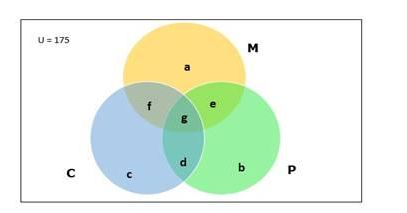# A class has 175 students. The following description gives the number of

Question:

A class has 175 students. The following description gives the number of students one or more of the subjects in this class: mathematics 100, physics 70, chemistry 46, mathematics and physics 30; mathematics and chemistry 28; physics and chemistry 23; mathematics, physics and chemistry 18. Find

(i) how many students are enrolled in mathematics alone, physics alone and chemistry alone,

(ii) The number of students who have not offered any of these subjects.

Solution:

Given:

- Number of students in class $=175$

- Number of students enrolled in Mathematics $=100$

- Number of students enrolled in Physics $=70$

- Number of students enrolled in Chemistry $=46$

- Number of students enrolled in Mathematics and Physics $=30$

- Number of students enrolled in Physics and Chemistry $=23$

- Number of students enrolled in Mathematics and Physics $=28$

- Number of students enrolled in all three subjects $=18$

To find:

(i) Number of students enrolled in Mathematics alone, Physics alone and Chemistry alone

Venn diagram:Number of students enrolled in Mathematics $=100=n(M)$

Number of students enrolled in Physics $=70=n(P)$

Number of students enrolled in Chemistry $=46=n(C)$

Number of students enrolled in Mathematics and Physics

$=30=n(M \cap P)$

Number of students enrolled in Mathematics and Chemistry

$=28=n(M \cap C)$

Number of students enrolled in Physics and Chemistry

$=23=n(P \cap C)$

Number of students enrolled in all the three subjects

$=18=n(M \cap P \cap C)=g$

We have

$n(M \cap P)=e+g$

$30=e+18$

$e=30-18=12$

$n(M \cap C)=f+g$

$28=f+18$

$f=28-18=10$

$n(P \cap C)=d+g$

$23=d+18$

$d=23-18=5$

a = Number of students enrolled only in Mathematics

b = Number of students enrolled only in Physicsc = Number of students enrolled only in Chemistry

We have,

$M=a+e+f+g$

$100=a+12+10+18$

$a=100-40$

$a=60$

Therefore,

Number of students enrolled only in Mathematics = 60

$P=b+e+d+g$

$70=b+12+5+18$

$b=70-35$

$b=35$

Therefore,

Number of students enrolled only in Physics = 35

$C=c+f+d+g$

$46=c+10+5+18$

$c=46-33$

$c=13$

Therefore,

Number of students enrolled only in Chemistry = 13

(ii) Number of students who have not offered any of these subjects

Number of students who have not offered any of these subjects

$=175-\{n(M)+n(P)+n(C)-n(M \cap P)-n(M \cap C)-n(P \cap C)+n(M \cap P \cap C)\}$

$=175-(100+70+46-30-28-23+18)$

$=175-153$

$=22$

Therefore

Number of students who have not offered any of these subjects = 22